# Design of Control System Using Online Tuning of PI Controllers for Three-Phase Active Front End Neutral Point Clamped Three-Level Converter

Design of Control System Using Online Tuning of PI Controllers for Three-Phase Active Front End Neutral Point Clamped Three-Level Converter
Electrical Engineering Department, Maulana Azad National Institute of Technology, Bhopal 462003, India

Corresponding Author Email:
amitojh@manit.ac.in
Page:
869-882
|
DOI:
https://doi.org/10.18280/jesa.530613
4 August 2020
|
Accepted:
2 December 2020
|
Published:
23 December 2020
| Citation

OPEN ACCESS

Abstract:

In conventional DC link AC-AC converter system, uncontrolled front end converter has inherent problem of power quality. To overcome the problem associated with uncontrolled front end converter, multilevel front end converter will be good choice for high power applications. In the paper, unity power factor control system (UPC) for active front end (AFE) neutral point clamped (NPC) 3-level converter is presented. PI controllers are used in UPC and for tuning of PI controllers it is essentially required to know the different time constants with their gains exactly for evaluating the optimized controller parameters for steady operation of the NPC rectifier. In this paper a simplified and accurate method of online tuning of PI controllers in UPC is derived and implemented for three phase AFE NPC 3-level converter. Complete design procedure for the design of control system for AFE NPC 3-level is also presented in this paper. Based on developed model, simulations are carried in MATLAB/SIMULIK environment. Experimentation results confirm the simulation results.

Keywords:

PI controllers, 3-level converter, signal constraint, Total Harmonic Distortion (THD), MATLAB/SIMULINK

1. Introduction

Active front end converters have been widely used in variable speed drives . Uncontrolled device or SCRs based converters draws currents rich in harmonics and reactive component of the current from the source creates the issue of power quality [2, 3]. High power factor converters became the inherent part of AC-DC conversion [4-8] an effectively used to overcome these issues and for improved power quality. But these high-power factor converters utilize high voltage rating devices and they are having issues of high voltage stress across the device, dv/dt, high common mode voltage, high switching frequency etc. [9-12]. The performance of multilevel converter is excellent in terms of improved power quality, less ripple in output voltage, less voltage stress and low electromagnetic interference with neighbouring communication lines as compared to high power factor converters [13-26]. Amongst the conventional topologies of multilevel structure, Diode-clamped multilevel converter is used for power conditioning now a days [9-11].

A simplified current controlled controller is designed and developed for controlling output voltage and for maintaining the power factor at unity. The controller is comprising of PI controllers and tuning of PI controllers which is easy to implement in real time application is still brain storming research for researchers. For tuning of PI controllers there so many well-known optimisation techniques like Artificial Neural Network, Genetic Algorithm, Particle Sworn Optimization etc. are available for getting optimised controller parameters [27, 28]. These optimising techniques have their own advantages and disadvantages and provide solution based on available mathematical model of the system. Another aspect which ensures the accuracy of the solution provided by these techniques is the constraints defined for getting desired output.

Tuning of converters requires the optimization of controller parameters for defined constraints and stable operation of the system. Therefore, an appropriate optimization technique is required to use for the tuning of controllers as almost, all are doing. The available technique till now is system dependent and has their own advantages and disadvantages [29-31]. Also, there is serious issue of real time implementation with evaluated tuned parameters of the controller.

In the present scenario, almost all simulations are carried out in the MATLAB/SIMULINK environment because the same Simulink model can be used with sophisticated controller like dSPACE 1104,1103, microLabbox etc. as well as with low cost controllers like STM32 microcontroller for real time implementation.

Therefore, there is an attempt to use available optimization software with MATLAB/SIMULINK environment to tune the controllers for the design and development of complex system. In this paper the author designed a complex 3-phase AFE NPC 3-level converter with controller using optimization software available in Simulink Design Optimization library. The proposed system is also developed in the laboratory and confirms that it is easier way to tune the controllers with more accuracy. The term online is used because tuning technique used is a part of MATLAB/SIMULINK environment and it optimised the controller parameters by communicating the Simulink model internally. This will defiantly save the time as well as digital space requirement and improve the performance of the system.

The whole paper is organised in seven (07) sections. In section 2, survey of literatures related to the tuning of controllers is carried out for establishing the appropriate research gap. There is description about the 3-phase AFE NPC 3-level converter in section 3. The complete design of controller is presented in section 4. Section 5 deals with the simulation results of proposed tuning technique for the controller of 3-phase AFE NPC 3 level converter. The hardware results for the validation of simulation results of the proposed system are presented in section 6 followed by conclusion in section 7.

2. Tuning of Controllers: A Survey

There are many tuning methods are proposed till now to tune the controller for considerable improvements in performance of controller. The merits and de-merits of some commonly used tuning method are discussed in Table 1.

There are literatures related to the tuning methods of PI controllers [27-31]. The objective all are same to assess the relative control effort and robustness of these PI controllers when tuned for the same level of performance. Some methods with simple formulas use little information of process dynamics to obtain moderate performance, however it often need to retune by trial and error depend on those results. More sophisticated tuning method can get rise to considerable improvements in performance, but they are also more demanding computationally and depend on more information of process dynamics.

The above analysis clearly shows that the performance of the controller depends on the optimization of the parameters. The choice of method should be based on the characteristics of the process and performance requirements. Therefore, author attempted to use optimization software available with MATLAB/SIMULINK library to tune the PI controllers to maintain the balance between characteristics of the process and performance requirement. The feature of online tuning method using signal constraint is that its compliance most of requirement for tuning of controller without much sacrificing the performance of the controller. This is because it is inbuilt optimization software in MATLAB/SIMULINK and in most of the sophisticated controller like dSPACE 1104, 1103, microlabbox etc., Simulink model can effectively build and run. Therefore, the communication of the Simulink model with the inbuilt optimization software is very fast. Also, it minimizes the complexity of the controller which is important from the practical implementation point of view.

2.1 Tuning of PI controllers using Signal Constraint block

In this section the technical aspect of the tuning of controller using optimization software available in MATLAB/SIMULINK library has been explained. For the tuning of a controller following parameters of the signal has to be taken into the consideration.

1. Rise time
2. Maximum overshoot
3. Settling time

When optimization of parameters of a Simulink model to meet time-domain design requirements attempted, Simulink Design Optimization software automatically converts the requirements into a constrained optimization problem and then solves the problem using optimization techniques. The constrained optimization problem iteratively simulates the Simulink model, compares the results of the simulations with the constraint objectives, and uses optimization methods to adjust tuned parameters to better meet the objectives.

Table 1. Comparison of different methods for tuning the controllers

 Method Key Features Disadvantages Ziegler-Nichols (Z-N) method It is most commonly used method for tuning and has very strong impact on controller. The tuning methods are based on a step response experiment and on a frequency response experiment. The main drawback of the method is the use of information for characterization of process dynamics with poor damping and poor robustness. Haalman and λ -Tuning method In this method the process poles and zeros are canceled by poles and zeros in the controller. With this feature a specified closed-loop transfer function can be obtained that gives the desired performance which the key feature of the controller. But the issue with the cancellation is the chance of uncontrollable modes in the closed-loop system and the response to load disturbances may be poor when the canceled poles are slow in comparison with the dominant poles Pole placement method This method is preferably used for low order system like first or second order system. For higher order system the controller becomes complex. Graphic tuning method This is the graphical method and most intuitive design method. The tuning method is based on finding the centroids of stability regions in the controller parameter space, considering a point which is situated away from the boundaries of the stability region, and consequently the controller, which is tuned based on such a point, will be nonfragile. The main disadvantage of this method is it is very time-consuming and neglect of the other important performance indicators. M -constrained integral gain optimization (MIGO) It is non-convex optimization method to obtain the controller parameters This optimization can be solved by some iterative algorithm such as Newton-Raphson or Genetic algorithms. The issue with this method is that it requires considerable amount of computational capacity. AMIGO and Kappa-Tau tuning method AMIGO is an approximation of MIGO and can be used for wide range of system. Processes with lag dominated dynamics, balanced lag and delay or dominated dynamics can use this method to tuning controller to get an acceptable performance. Kappa-Tau tuning method is very similar to the MIGO method. The different is only the Kappa-Tau tuning method is an approximation to pole placement. The performance of the controller using this method is inferior as compared to MIGO Internal model principle (IMC) method The key feature of this method is that it has only one user-defined tuning parameter, which attracts many industrial users. The excellent set-point response can be obtained by the IMC, but the response to load disturbances is often poor.
The software first formulates the problem for parameter optimization. For each optimization algorithm, the software formulates one of the following types of minimization problems
1. Feasibility
2. Tracking
3. Mixed Feasibility and Tracking.
• Feasibility Problem and Constraint Formulation

Feasibility means that the optimization algorithm finds parameter values that satisfy all constraints to within specified tolerances but does not minimize any objective or cost function in doing so. In the following Figure 1, x1, x3, and xn represent a combination of parameter values P1 and P2 and are feasible solutions because they do not violate the lower bound constraint.

## 1.pngFigure 1. Feasibility response

In a Simulink model, one can constrain a signal by specifying lower and upper bounds in a block, as shown in the following Figure 2.

## 2.pngFigure 2. Appling the constraints on signal using Signal Constraint block

These constraints are piecewise linear bounds. A piecewise linear bound ybnd with n edges can be represented as:

{{y}_{bnd}}(t)=\left\{ \begin{align} & {{y}_{1}}(t)\,\,\,\,\,\,\,{{t}_{1}}\le t\le {{t}_{2}}\,\,\,\,\,\,\, \\ & {{y}_{2}}(t)\,\,\,\,\,\,{{t}_{2}}\le t\le {{t}_{3}} \\ & \vdots \,\,\,\,\,\,\,\,\,\,\,\,\,\,\,\,\,\,\,\,\,\,\,\,\,\,\vdots \\ & {{y}_{n}}(t)\,\,\,\,\,\,{{t}_{n}}\le t\le {{t}_{n+1}} \\ \end{align} \right.           (1)

The software computes the signed distance between the simulated response and the edge. The signed distance for lower bounds is:

c=\left[ \begin{align} & \underset{{{t}_{1}}\le t\le {{t}_{2}}}{\mathop \max }\,\,\,\,\,\,\,\,\,\,\,{{y}_{bnd}}-{{y}_{\sin }} \\ & \underset{{{t}_{2}}\le t\le {{t}_{3}}}{\mathop \max }\,\,\,\,\,\,\,\,\,\,\,{{y}_{bnd}}-{{y}_{\sin }} \\ & \underset{{{t}_{n}}\le t\le {{t}_{n+1}}}{\mathop \max }\,\,\,\,\,\,\,\,{{y}_{bnd}}-{{y}_{\sin }} \\ \end{align} \right]                     (2)

where, ysim is the simulated response and is a function of the parameters being optimized.

The signed distance for upper bounds is:

c=\left[ \begin{align} & \underset{{{t}_{1}}\le t\le {{t}_{2}}}{\mathop \max }\,\,\,\,\,\,\,\,\,\,\,{{y}_{\sin }}-{{y}_{bnd}} \\ & \underset{{{t}_{2}}\le t\le {{t}_{3}}}{\mathop \max }\,\,\,\,\,\,\,\,\,\,\,{{y}_{\sin }}-{{y}_{bnd}} \\ & \underset{{{t}_{n}}\le t\le {{t}_{n+1}}}{\mathop \max }\,\,\,\,\,\,\,\,{{y}_{\sin }}-{{y}_{bnd}} \\ \end{align} \right]                     (3)

If all the constraints are met (c ≤ 0) for some combination of parameter values, then that solution is said to be feasible. In the following Figure 3, x1 and x3 are feasible solutions.

## 3.pngFigure 3. Possible feasible solutions using Signal Constraint block

When the model has multiple Signal Constraint blocks or vector signals feeding a Signal Constraint block, the constraint vector is extended with the constraint violations for each signal and bound:

$C=\left[ {{c}_{1}};{{c}_{2}};\cdots ;{{c}_{n}} \right]$         (4)

• Tracking Problem

In addition to lower and upper bounds, you can specify a reference signal in a Signal Constraint block, which the Simulink model's output can track. The tracking objective is a sum-squared-error tracking objective.

The reference signal can be specified as a sequence of time-amplitude pairs:

${{y}_{ref}}({{t}_{ref}}),{{t}_{ref}}\in \left\{ \,\,{{T}_{ref0}},{{T}_{ref1}},\cdots ,{{T}_{refN}} \right\}$          (5)

The software computes the simulated response as a sequence of time-amplitude pairs:

${{y}_{\sin }}({{t}_{\sin }}),{{t}_{\sin }}\in \left\{ \,\,{{T}_{\sin 0}},{{T}_{\sin 1}},\cdots ,{{T}_{\sin N}} \right\}$         (6)

where, some values of tsim may match the values of tref.

A new time base, tnew, is formed from the union of the elements of tref and tsim. Elements that are not within the minimum-maximum range of both tref and tsim are omitted:

${{t}_{new}}=\left\{ \,t:{{t}_{\sin }}\cap {{t}_{ref}} \right\}$        (7)

Using linear interpolation, the software computes the values of yref and ysim at the time points in tnew and then computes the scaled error:

$e({{t}_{new}})=\frac{({{y}_{\sin }}({{t}_{new}})-{{y}_{ref}}({{t}_{new}}))}{\underset{{{t}_{new}}}{\mathop \max }\,\left| {{y}_{ref}} \right|}$           (8)

Finally, the software computes the weighted, integral square error:

$f=\int{w(t)e{{(t)}^{2}}dt.}$       (9)

When the model has multiple Signal Constraint blocks or vector signals feeding a Signal Constraint block, the tracking objective equals the sum of the individual tracking integral errors for each signal:

$f=\int{w(t)e{{(t)}^{2}}dt.}$      (10)

The above explanation clearly explains how the optimization of parameters is carried out using optimization software and Signal Constraint block. The tuning of the controller using this method having so many advantages but it has some limitations also like optimization software is inbuilt and has no access. Therefore, changes/modifications are not possible and for defining the constraints on signal only GUI of the software can be used. Therefore, the accuracy of the tuning is depending on the user ability for defining the constraint on the signal using GUI. The use of the technique is restricted to platforms where Simulink models can be built and run.

3. AFE NPC 3-Level Converter

Figure 4 shows that there are four devices are required for each phase and clamping diodes are used for clamping in each leg. Three phase supply is given to 3-level converter through inductor. The midpoint of dc link capacitors on the output side and each phase clamping diodes is connected to the neutral on the supply side. Resistance R1 and R2 are connected as shown in Figure 4 for loading the converter.

The power devices (Sx1, Sx2, Sx1 and Sx2, x=a, b, c) in each leg are controlled independently and constraints for the connected devices so as to avoid the devices to conduct simultaneously is defined below:

${{S}_{xi}}+S_{xi}^{'}=1$       (11)

where, x = a, b, c and i = 1, 2.

$S_{x i}$= 1 (switch $S_{x i}$ is turned on) or $S_{x i}$= 0 (switch $S_{x i}$ is turned off). Hence, the following is the equivalent switching function of the 3-level converter:

{{T}_{Sx}}=\left\{ \begin{align} & \,\,\,\,1\,\,\,\,\,if\,\,{{S}_{x1}}={{S}_{x2}}=1\,\, \\ & \,\,\,\,0\,\,\,\,if\,\,S_{x1}^{'}={{S}_{x2}}=1\,\, \\ & -1\,\,\,\,\,if\,\,S_{x1}^{'}=S_{x2}^{'}=1\,\, \\ \end{align} \right.          (12)

The above switching function shows that there are three possible modes of operation. The simulation of 3-phase AFE NPC 3-level converter is carried out in MATLAB/SIMULIK environment and results are presented in section 5.

4. Design of Controller (UPC)

A controller is required to operate 3-level converter at unity power factor for improved power quality. The Figure 5 shows the control system for operating 3-level converter at unity power factor and termed as unity power factor controller (UPC). The UPC comprises of voltage controller, neutral point potential regulator (NPP) and current controller for which online tuning of PI controllers is explained.

## 4.pngFigure 4. 3-Phase AFE NPC 3-level converter

## 5.pngFigure 5. Schematic of Unity Power Factor Controller (UPC)

Van, Vbn and Vcn are the carrier based unipolar PWM line-to-neutral voltages produced at input side of the converter . Three-unit sinusoidal voltages are generated using the phase locked loop (PLL) circuit which is synchronized with the AC source voltages. The output of the voltage controller comprising of PI controller to minimize the error between two dc link voltages, is the amplitude of the input current commands (Im) which is multiplied by the generated unit sinusoidal voltages to generate reference supply currents (isa*, isb*, isc*).

The generated reference current is then added to output of NPP regulator. The resultant is compared with actual currents and processed through PI controllers. Control or reference voltages are generated by comparing the output of PI controller and output of PLL [29, 30]. Gating signals are obtained for the devices by comparing these reference/control voltage signals with triangular carrier waves.

4.1 Design of current controller

The line current commands which are defined in equation 13 are compared with sensed actual currents and the error is fed to current controllers which comprises of PI controllers for tracking the source current command.

\left. \begin{align} & i_{a}^{*}(t)={{I}_{m}}\sin (\omega t) \\ & i_{b}^{*}(t)={{I}_{m}}\sin \left( \omega t-{}^{2\pi }/{}_{3} \right) \\ & i_{c}^{*}(t)={{I}_{m}}\sin \left( \omega t+{}^{2\pi }/{}_{3} \right) \\ \end{align} \right\}                  (13)

Neglecting the high-frequency switching terms, source voltage of phase ‘a’ is define below.

${{V}_{sa}}={{L}_{sa}}\frac{d{{i}_{sa}}}{dt}+{{V}_{cona}}$       (14)

where, $V_{\text {cona}}$ is defined as modulated control signal of PWM based converter from the proposed closed loop current controller. The multi carrier-based SPWM scheme is used for generating the required gating signals as shown in Figure 6 (a & b).

The switching signals for the power switches derived from the SPWM based control system and gating signals can be defined as:

{{T}_{c}}=\left\{ \begin{align} & \,\,\,\,1\,\,\,\,\,if\,\,{{v}_{cona}}\,>{{v}_{t1}}\,\, \\ & \,\,\,\,0\,\,\,\,if\,\,{{v}_{t1}}\,>{{v}_{cona}}\,>\,{{v}_{t2}} \\ & -1\,\,\,\,if\,\,\,{{v}_{t2}}\,>\,{{v}_{cona}} \\ \end{align} \right.                      (15)

Hence switching function can be written as:

\left. \begin{align} & {{S}_{a1}}=\frac{{{T}_{c}}({{T}_{c}}+1)}{2} \\ & S_{a1}^{'}=1-{{S}_{a1}} \\ & S_{a2}^{'}=\frac{{{T}_{c}}({{T}_{c}}+1)}{2} \\ & {{S}_{a2}}=1-S_{a2}^{'} \\ \end{align} \right\}          (16)

In the first half cycle of the control signals Vcona, the line current is controlled by turning on or off the power switch Sa1 while the switch Sa2 is remain turned on during the half cycle for phase ‘a’. And in the second half cycle the line current is controlled by turning on or off Sa2, and Sa1 is turned off. The phase voltage Van generated on AC side is three level i.e V0/2, 0, and - V0/2.

4.1.1 Tuning of current controller

The performance of a 3-level converter depends on the current control strategy. As compared to open loop converters, following are the advantages of current-controlled PWM (CC-PWM) converter.

• Instantaneous current waveform Control with high accuracy;
• Protection against peak current;
• Dynamic repose is extremely good;
• Effects of Compensation due to change in load parameter (R and L);
• AC-side voltage and DC-bus changes Compensation.

## 6.pngFigure 6. (a) SPWM based control pulses generation; (b) Switching pattern

The PWM based current control techniques can be classified as linear and non-linear controllers .

By implementing this concept, it is possible to take the advantages of open loop modulators like space vector PWM, sinusoidal PWM etc. for constant switching frequency with improved power quality and optimal gating pattern. It is also possible to design controller independently with open loop testing with ease. The performance of the multilevel converter depends on the type of reference frame is used for implementing the PI controllers. Literature shows  the synchronous reference frame gives better steady state response as compared to stationary reference frame.

Therefore, PI controllers in synchronous reference frame is designed and implemented in this paper. The block diagram of decoupled controller in synchronous reference frame for multilevel rectifier is shown in Figure 7.

## 7.pngFigure 7. Block diagram of overall control of 3-Phase 3-level converter

The tracking capability of current controller can be improved by decoupling the d and q axes which reduce the synchronous reference-frame current control plant to a first-order delay.

By doing so it is simple to analysis and allow to derive analytical expressions for the parameters of current controller.

Figure 8 shows the block diagram of simplified current control close loop in the synchronous reference frame.

As shown in the Figure 8, the gains and time-constants of various elements are as follows:

 Ts Sampling time (Ts = 1/fs), UL Disturbance voltage Kcp PI current controller’s Proportional gain Tci PI current controller’s Time-constant Kc Gain of PWM rectifier (Kc = 1) TF Time delay of filter Tmp Processing time of DSP To Dead-time (To = Ts / 2) of Power converter Tf Feedback Time delay filter and sampling

The following are the assumptions made

• The cross-coupling effect due to inductance between d and q axes is neglected.
• Power converter dead-time which includes processing and sampling time is approximated by a first-order inertia element

${{e}^{-sT}}\approx \frac{1}{1+s{{T}_{o}}}$       (17)

• The sum of small time constants is defined as:

$\tau ={{T}_{\mu p}}+{{T}_{o}}+{{T}_{f}}$         (18)

## 8.pngFigure 8. Block diagram of synchronous current controller

The block diagram simplified synchronous current controller is shown in Figure 9 considering UL=0.

## 9.png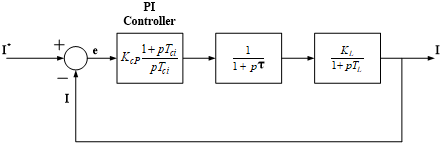Figure 9. Block diagram of simplified of synchronous current controller

Hence, the open loop transfer function can be expressed as given equation:

${{G}_{o}}(p)={{K}_{cp}}\left( \frac{1+p{{T}_{ci}}}{p{{T}_{ci}}} \right)\,\left( \frac{1}{1+p\tau } \right)\,\left( \frac{{{K}_{L}}}{1+p{{T}_{L}}} \right)$       (19)

${{G}_{o}}(p)={{K}_{cp}}\left( 1+\frac{1}{p{{T}_{ci}}} \right)\,\left( \frac{{{K}_{L}}}{\left( 1+p\tau \right)\,\left( 1+p{{T}_{L}} \right)} \right)$      (20)

And the characteristic equation is defined as:

$1+{{G}_{o}}(p)\,{{H}_{o}}(p)=0$    (21)

$1+{{K}_{cp}}\left( 1+\frac{1}{p{{T}_{ci}}} \right)\,\left( \frac{{{K}_{L}}}{{{p}^{2}}\tau {{T}_{L}}+p{{T}_{L}}+p\tau +1} \right)=0$        (22)

The Eq. (22) shows that Synchronous Current Controller is of third order and the optimised parameter for the stable operation of 3-level converter can be found out using accurate available techniques.

Therefore, online tuning of PI controller is used to find the optimised parameter by using Signal Constraint block available in Simulink Design Optimisation library in MATLAB/Simulink environment.

For online tuning the Signal Constraint block is attached to the Simulink model for optimizing the model response to know inputs. Design optimization software in Simulink tunes the parameter of the model as per the applied constraint. The constraints are defined to track the reference signals. The constraints are applicable to vector and matrix-valued ports, in which case the signal bounds and reference signals apply to all entries of the signal/matrix. The optimising methods available in Signal Constraint block are gradient descent, pattern search and simplex search. The gradient descent method with basic gradient type is used to tune current controller for optimum performance of NPC rectifier. The Signal Constraint block is attached to synchronous current controller in the feedback loop as shown in Figure 10.

## 10.pngFigure 10. Simplified block diagram of current control loop

The step response of the current controller after completion of optimization process is shown in Figure 11.

## 11.png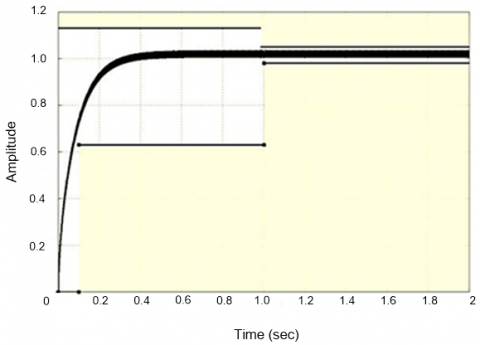Figure 11. Step response of synchronous current controller

And the optimised proportional gain (Kcp) and integral gain (Kci) are found 0.2 and 10 respectively. Thus, the selected transfer function of the PI current controller is given by:

$0.2\left[ \frac{(1+0.02\,p)}{0.02\,p} \right]$         (23)

4.2 Design of voltage controller

A voltage controller is used to maintain the constant DC voltage for balancing of power at AC and DC side. The error of reference voltage and sensed voltage is processed through PI controller to derive amplitude of the line current command (Im) which is given by

${{I}_{m}}={{k}_{p}}\Delta {{V}_{0}}+{{k}_{i}}\int{\Delta {{V}_{0}}}$        (24)

where, $\Delta V=V_{0}^{*}-V_{0}$ is voltage error, ${{V}^{*}}_{_{0}}$ is the reference DC link voltage and ${{V}_{0}}$ is sensed DC link voltage. The PLL is used to generate unit sinusoidal voltages can be written as:

\left. \begin{align} & {{V}_{sau}}(t)=\sin \omega t \\ & {{V}_{sbu(t)}}=\sin (\omega t+{}^{2\pi }/{}_{3}) \\ & {{V}_{scu(t)}}=\sin (\omega t-{}^{2\pi }/{}_{3}) \\ \end{align} \right\}                   (25)

4.2.1 Tuning of voltage controller

The voltage controller can be modelled and its block diagram is shown in Figure 12. Following are the various gains and time constants associated with voltage control loop.

 Tdv Feedback-loop delay due to sampling, processing time and feedback filter Kvp Proportional gain of voltage PI controller Tvi Time-constant of voltage PI controller C DC link capacitance

The inner current control loop is modelled with the first order transfer function Hci and given by-

${{H}_{ci}}=\frac{i}{{{i}^{*}}}=\frac{1}{1+p\,{{T}_{et}}}$         (26)

where, Tet = 2t.

Combining the two smallest time constant in Figure 8 (Tdv and 2t of Hci), the equivalent time constant Tev is obtained as:

${{T}_{ev}}={{T}_{dv}}+2\tau$       (27)

The simplified block diagram of Figure 12 is shown in Figure 13.

## 12.png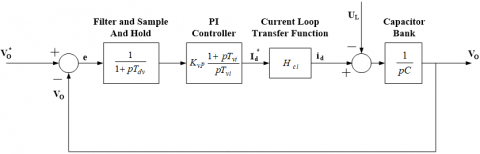Figure 12. Block diagram of voltage control loop

## 13.pngFigure 13. Simplified block diagram of voltage control loop

Assumption is made that the dc link voltage is equal and potential of neutral point is zero. Then the total dc link capacitance is:

$C=\frac{{{C}_{1}}(or\,{{C}_{2}})}{2}$      (28)

And the dc link voltage is evaluated as:

${{V}_{0}}=\frac{1}{C}\int{{{i}_{c}}dt\,=\frac{1}{C}\int{{{i}_{d}}dt}}$         (29)

The open loop transfer function is defined as:

$G(s)=\left[ \frac{{{K}_{vp}}(1+p\,{{T}_{vi}}}{p{{T}_{vi}}} \right]\,\left( \frac{1}{1+p{{T}_{ev}}} \right)\,\left( \frac{1}{pC} \right)$  (30)

Hence, the characteristic equation of voltage control loop is given by:

$1+G(s)\,H(s)=0$         (31)

i.e.

$1+\frac{{{K}_{vp}}\,(1+p{{T}_{vi}})}{p{{T}_{vi}}\,(1+p{{T}_{ev}})\,pC}=0$       (32)

## 14.png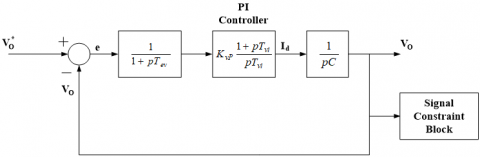Figure 14. Simplified block diagram of voltage control loop with Signal Constraint block

The characteristic equation shows that the system is of third order. The same Signal Constraint block has been used to evaluate PI controller parameters as explained in Section 4.1.1.

The Signal Constraint block is attached to output of voltage controller in feedback loop which acquires the output dc link voltage for the tuning of PI voltage controller as shown in Figure 14. The output of Signal Constraint block shows different curves for different values of proportional gain (Kvp) and integral gain (Kvi) of voltage controller as shown in Figure 15. The curve in black is the final step response of voltage controller for optimum values of proportional gain (Kvp) and integral gain (Kvi) of voltage controller.

## 15.pngFigure 15. Step response of synchronous voltage controller

The optimised proportional gain (Kvp) and integral gain (Kvi) for the obtained step response of synchronous voltage controller are 2 and 25 respectively. Hence, the transfer function of the PI voltage controller is given by:

$2\left[ \frac{(1+0.08\,p)}{0.08\,p} \right]$       (33)

4.3 Design of NPP regulator

NPP regulator is designed to control the neutral point potential and it can be done by using general law for the neutral point current as shown under (assuming $C_{1}=C_{2}$).

${{i}_{1}}\,=\,{{C}_{{}}}\frac{d{{V}_{c1}}}{dt}$     (34)

${{i}_{2}}\,={{C}_{{}}}\frac{d{{V}_{c2}}}{dt}$       (35)

Neutral point current is given by:

${{i}_{0}}=-\,{{i}_{1}}+{{i}_{2}}\,$     (36)

${{i}_{0}}=-C\frac{d({{V}_{c1}}-{{V}_{c2}})}{dt}$       (37)

The equation yields,

${{V}_{c1}}-{{V}_{c2}}=-\frac{1}{C}\int{{{i}_{0}}dt+cons\tan t}$      (38)

The Eq. (38) shows that i0 can be used to control NPP.

4.3.1 Tuning of NPP regulator

For tuning the NPP controller the procedure will remain the same as explained in 3.2.1. And the optimal parameters for tuned NPP controller are 2 for defined constraints.

5. Simulation Results

In this section, simulation results are presented based on designed UPC (Figure 5) for AFE NPC converter (Figure 6). The simulation study is carried out to evaluate the performance of AFE NPC 3-level converter under transient and steady state condition with online tuned parameters for UPC using Signal Constraint block of Simulink Design Optimisation library in MATLAB/Simulink environment. The simulation parameters and selected UPC parameters are given in Table 2 and Table 3 respectively.

Table 2. Simulation parameters for 3-level converter

 RMS Phase Voltage Vsa 3810 V Inductor Ls 30mH Resistance on AC Side R 0.8 Ω Voltage DC Side Vdc 11000 V Capacitance C1/ C2 100000 µF Load Resistance R1 || R1’ 60 Ω || 60 Ω R2 || R2’ 60 Ω || 60 Ω Switching Frequency fc 2050 Hz

The unity power factor operation of converter is the important criteria for the evaluation of performance of the converter. The result shown in Figure 16, confirms that the PI controllers of current controller are accurately tuned as supply current is in phase with supply voltage which is primary requirement of the UPC. The harmonic profile of phase ‘a’ line current is shown in Figure 17 and THD is well below the limit defined in IEEE519 standard i.e. 2.39%.

Table 3. Selected tuned controller for the converter

 Current Controller Parameters Kcp 0.2 Kci 10 Voltage Controller Parameters Kvp 2 Kvi 25 NPP Regulator Parameters Knp 2

## 16.pngFigure 16. Source voltage (Vsa) and line current (isa) of Phase ‘a’

## 17.pngFigure 17. Harmonic spectrum of line current (isa)

## 18.png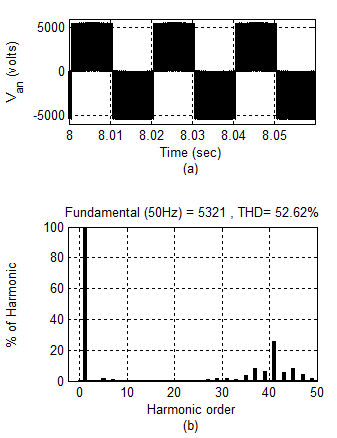Figure 18. AC side (a) phase voltage (Van) (b) Harmonic spectrum of phase voltage (Van)

## 19.pngFigure 19. AC side (a) Line-Line voltage (Vab) (b) Harmonic spectrum of line-line voltage (Vab)

## 20.png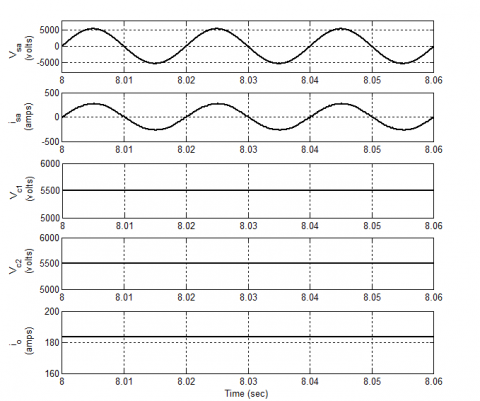Figure 20. Voltage (Vsa), current (isa), dc link capacitor voltages (Vc1 and Vc2) and load current (i0) under steady state condition

Another important performance index of 3-level converter is performance of converter under steady state. Therefore, AC side phase voltage and its THD profile and AC side line voltage with its THD profile has been observed and shown in Figure 18 and Figure 19 respectively. Also, the steady state phase voltage (Vsa), Line current (isa), dc link capacitor voltages (Vc1 and Vc2) and load current (i0) are shown in Figure 20. The result shows satisfactory operation of the converter under steady state condition.

The results shown in these Figures clearly show the stable operation of AFE NPC 3-level under steady state.

5.2 Performance under transient condition

The real testing of the PI controllers of UPC is the satisfactory performance of the converter under transient condition. Therefore, the dynamic behaviour of 3-level converter for tuned PI controllers has been observed under transient conditions.

DC link capacitor voltages Vc1 and Vc2 and Phase ‘a’ source current (isa) is shown in Figure 21. The result shows that Vc1 and Vc2 settled down quickly (within 10 cycles) and isa remains sinusoidal after sudden increase in load current.

## 21.png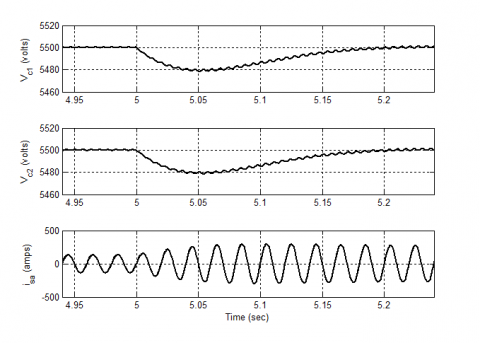Figure 21. Capacitor Voltages (Vc1 and Vc2) and current (isa) for increase in load at t = 5 sec

The dynamic response of the converter when load is suddenly reduced is also analysed. Figure 22 shows Vc1 & Vc2 and isa when load is reduced and it is observed that it settled down within 10 cycles due to proper tuning of PI controllers in the current and voltage controller.

## 22.pngFigure 22. Capacitor Voltages (Vc1 and Vc2) , and current (isa) for decrease in load at t = 10 sec

The simulation results under transient condition validate the optimised tuning parameters of PI controllers of UPC.

5.3 Neutral Point Potential (NPP) control

## 23.pngFigure 23. DC link capacitor voltages (Vc1 & Vc2) under unbalanced load

The working of NPP is also verified through simulation by introducing the unbalance load. The Vc1 & Vc2 are shown in Figure 23 which shows the effective working of NPP regulator when it is included at t=12 sec.

6. Experimental Results

A prototype is developed in the laboratory for validating the simulation results. The developed experimental setup comprises of following parts:

1. Power circuit of AFE NPC 3-level converter (IGBT rectifier and gate driver circuit),
2. Measurement of system parameters such as supply voltages, line currents and dc link voltages,
3. Implementation of control scheme through dSPACE DS1103 real time controller,
4. Interfacing DS1103 Master Bit I/O with the associated hardware.

Real time implementation is done for performing the experimentation on developed prototype. The parameters chosen for experimentation are given in Table 4.

Table 4. Experimental parameters for NPC AFE 3-Level converter

 RMS Line Voltage VLL 50 V Inductor (source) Ls 7.73mH Resistance (AC Link) R 0.4 Ω DC Link Parameters Voltage (DC Link) Vdc 80 V DC Link Capacitors C1 and C2 2200 µF Load Parameters Loading Resistances (R1 and R2) 15 Ω Switching Frequency fc 2050

The experimental results show that supply voltage and current and in phase (Figure 24) which validate the simulation results. The harmonic spectrum of isa and Vab shown in Figure 25 (a & b) and shows that the THD in supply current is only 2.4% and verify the simulation results.

## 24.png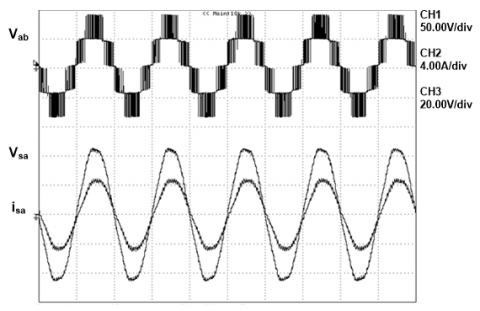Figure 24. Voltage (Vab) at supply end, phase voltage (Vsa) and current (isa)

X - axis: Time – 10ms/div, Y axis: Vab - 50 V/div, Vsa – 20V/div and isa – 4A/div

## 25.pngFigure 25. Harmonic spectrum of (a) Line current (isa) and (b) Line voltage harmonic spectrum at supply end (Vab)

The phase voltage (Van) at supply end is shown in Figure 26 under steady state condition.

## 26.pngFigure 26. Voltage (Van) at supply end of 3-level converter under steady state condition

X - axis: Time –10ms/div, Y - axis: Van - 20 V/div

The dc link capacitors voltages Vc1 and Vc2 under steady state condition are shown in Figure 27. This validates effective working of UPC maintaining desired dc link voltage.

## 27.pngFigure 27. Capacitor voltages (Vc1 and Vc2) of 3-level converter under steady state condition

X axis: Time- 10ms/div, Y axis: Vc1 and Vc2 - 20 V/div

6.2 Performance under Transient Condition

For validating the dynamic response of the converter under transient condition, the load is suddenly changed and response of Vc1 and Vc2 and isa has been recorded (Figure 28 and Figure 29). The results confirmed that the PI controllers in UPC is effectively tuned and able to regulate dc link voltage quickly at desired value under transient condition. Therefore, the tuned parameters obtained from the optimization software are the required parameters for the real time implementation of the proposed system.

## 28.pngFigure 28. Capacitor voltages (Vc1 and Vc2) and current (isa) when load is increased

X - axis: Time – 1s/div, Y - axis: Vc1 and Vc2 - 20 V/div, isa – 4 A/div

## 29.png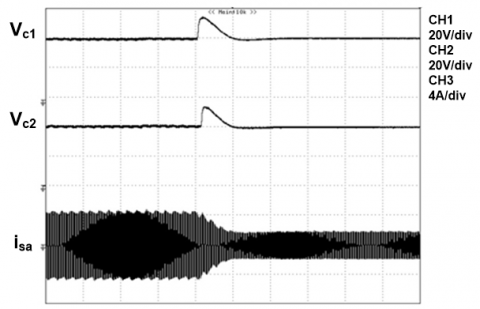Figure 29. Capacitor voltages (Vc1 and Vc2) and current (isa) when load is decreased

X - axis: Time – 1s/div, Y- axis: Vc1 and Vc2 - 20 V/div, isa – 4 A/div

6.3 NPP control

## 30.pngFigure 30. NPP variation in NPC AFE 3-level converter

A neutral point potential regulator is used in UPC to control the NPP variations. To verify its effective working experimentally, NPP regulator has been included in UPC. The NPP variations are shown in Figure 30. The experimental result also confirms that when NPP regulator is included in the controller, the variations has been considerably reduced.

7. Conculsion

Design of unity power factor controller is presented in this paper for active front end neutral point clamped 3-level converter. A simplified and accurate online tuning of PI controllers is derived and implemented for AFE NPC 3-level converter. Complete modelling of UPC controller is presented and simulation is carried out for the verification of derived online tuning method of PI controllers in UPC. The simulation result with online tuning of PI controllers in UPC confirms the satisfactory performance of AFE NPC 3-level converter under steady state and transient condition. A laboratory prototype is also developed for implementation of UPC with online tuning for AFE NPC 3-level converter. Experimental results validate the simulation results and confirm the easier and effective implementation of proposed online tuning method in real time application.

References

 Rashid, M.H. (2001). Power Electronics Handbook. Academic Press, New York.

 Sen, P.C. (1998). Power Electronics, Tata McGraw Hill Pub.

 Akagi, H. (1996). New trends in active filters for power conditioning, industry applications. IEEE Transactions on Industry Applications, 32(6): 1312-1322. https://doi.org/10.1109/28.556633

 El-Habrouk, M., Darwish, M.K., Mehta, P. (2000). Active power filters: A review, electric power applications. IEE Proceedings, 147(5): 403-413. https://doi.org/10.1049/ip-epa:20000522

 Jain, S.K., Agrawal, P., Gupta, H.O. (2002). Fuzzy logic controlled shunt active power filter for power quality improvement. Electric Power Applications, IEE Proceedings, 149(5): 317-328. https://doi.org/10.1049/ip-epa:20020511

 Hengchun, M., Fred, C., Lee, Y., Boroyevich, D., Silva, H. (1997). Review of high-performance three-phase power-factor correction circuits. IEEE Trans. on Industrial Electronics, 44(4): 437-446. https://doi.org/10.1109/41.605616

 Rodriguez, J.R., Dixon, J.W., Espinoza, J.R., Pontt, J., Lezana, P. (2005). PWM regenerative rectifiers: State of the art. IEEE Trans. on Industrial Electronics, 52(1): 5-22. https://doi.org/10.1109/TIE.2004.841149

 Singh, B., Singh, B.N., Chandra, A., Al-Haddad, K., Pandey, A., Kothari, D.P. (2004). A review of three-phase improved power quality AC-DC converters. IEEE Trans. on Industrial Electronics, 51(3): 641-660. https://doi.org/10.1109/TIE.2004.825341

 Marchesoni, M., Tenca, P. (2002). Diode-clamped multilevel converters: A practicable way to balance dc-link voltages. IEEE Trans. on Industrial Electronics, 49(4): 752-765. https://doi.org/10.1109/TIE.2002.801237

 Midavaine, H., Moigne, P.L., Bartholomeus, P. (1996). Multilevel three-phase rectifier with sinusoidal input currents. PESC Record. 27th Annual IEEE Power Electronics Specialists Conference, Baveno, Italy, pp. 1595-1599. https://doi.org/10.1109/PESC.1996.548794

 Zhou, J.H., Meng, Y.Q., Li, Z.X., Liu, K. (2008). Research on control method of three-level NPC voltage source rectifier. 2008 IEEE Vehicle Power and Propulsion Conference, Harbin, 2008, pp. 1-6. https://doi.org/10.1109/VPPC.2008.4677718

 Lai, J.S., Peng, F.Z. (1996). Multilevel converters: A new breed of power converters. IEEE Transactions on Industry Applications, 32(3): 509-517. https://doi.org/10.1109/28.502161

 Franquelo, L.G., Rodriguez, J., Leon, J.I., Kouro, S., Portillo, R., Prats, M.A.M. (2008). The age of multilevel converters arrives. Industrial Electronics Magazine, IEEE, 2(2): 28-39. https://doi.org/10.1109/MIE.2008.923519

 Gupta, K.K., Jain, S. (2013). A multilevel Voltage Source Inverter (VSI) to maximize the number of levels in output waveform. International Journal of Electrical Power & Energy Systems, 44(1): 25-36. https://doi.org/10.1016/j.ijepes.2012.07.008

 Thamizharasan, S., Baskaran, J., Ramkumar, S., Jeevananthan, S. (2013). A new dual bridge multilevel dc-link inverter topology. International Journal of Electrical Power & Energy Systems, 45(1): 376-383. https://doi.org/10.1016/j.ijepes.2012.09.024

 Bhat, A.H., Agarwal, P. (2006). An improved performance three-phase neutral-point clamped rectifier with simplified control scheme. IEEE International Symposium on Industrial Electronics, pp. 1019-1024. https://doi.org/10.1109/ISIE.2006.295776

 Kanaan, H.Y., Al-Haddad, K., Fnaiech, F. (2003). DC load unbalance and mains disturbances effects on a three-phase three-switch three-level boost rectifier. 2003 IEEE International Symposium on Industrial Electronics (Cat. No.03TH8692), Rio de Janeiro, Brazil, 2003, pp. 1043-1048. https://doi.org/10.1109/ISIE.2003.1267967

 Shu, Z., He, X., Wang, Z., Qiu, D., Jing, Y. (2013). Voltage balancing approaches for diode-clamped multilevel converters using auxiliary capacitor-based circuits. in IEEE Transactions on Power Electronics, 28(5): 2111-2124. https://doi.org/10.1109/TPEL.2012.2215966

 Josep, P., Boroyevich, D., Rafael, P. (2002). New feed forward space-vector PWM method to obtain balanced AC output voltage in a three-level neutral-point-clamped converter. IEEE Trans. on Industrial Electronics, 49(5): 1026-1034. https://doi.org/10.1109/TIE.2002.803207

 Ishida, T., Matsuse, K., Sugita, K., Huang, L.P., Sasagawa, K. (2000). DC voltage control strategy for a five-level converter, Power Electronics. in IEEE Transactions on Power Electronics, 15(3): 508-515. https://doi.org/10.1109/63.844511

 Olorunfemi, O., Konduru, S. (2005). A discontinuous carrier based PWM modulation method for the control of neutral point voltage of three-phase three-level diode clamped converters. 2005 IEEE 36th Power Electronics Specialists Conference, Recife, 2005, pp. 1652-1658. https://doi.org/10.1109/PESC.2005.1581852

 Chaturvedi, P., Ojha, A., Jain, S., Mittal, A. (2016). Unity power factor controller for neutral point clamped active front end converter with DC voltage balancing. 2016 IEEE International Conference on Industrial Technology (ICIT), Taipei, pp. 384-389. https://doi.org/10.1109/ICIT.2016.7474783

 Ojha, A., Chaturvedi, P.K., Mittal, A., Jain, S. (2017). Neutral point potential control for three phase 3-level neutral point clamped active front end converter. International Journal on Electrical Engineering and Informatics, 9(2): 342-363. https://doi.org/10.15676/ijeei.2017.9.2.10

 Ojha, A., Chaturvedi, P.K., Mittal, A., Jain, S. (2016). Carrier based common mode voltage reduction techniques in neutral point clamped inverter based AC-DC-AC drive system. Journal of Power Electronics, 16(1): 142-152. https://doi.org/10.6113/JPE.2016.16.1.142

 Janardhan, K., Mittal, A., Ojha, A. (2020). A symmetrical multilevel inverter topology with minimal switch count and total harmonic distortion. Journal of Circuits, Systems and Computers. Article in Press, 29(11): 2050174. https://doi.org/10.1142/S0218126620501741

 Tandekar, J., Ojha, A., Jain, S. (2019). Five-Level cascaded H-bridge MLC-based shunt active power filter for active harmonics mitigation in distributed network. Journal of Circuits, Systems and Computers, 28(2): 1950035. https://doi.org/10.1142/S021812661950035X

 Tandekar, J., Ojha, A., Das, S., Swarnkar, P., Jain, S. (2018). SEIG-based renewable power generation and compensation in MVDC ship power system. International Transactions on Electrical Energy Systems, 29(4). https://doi.org/10.1002/etep.2785

 Tandekar, J., Ojha, A., Jain, S. (2019). SEIG-based renewable generation for MVDC ship power system with improved power quality. Electric Power Components and Systems, 47(1-2): 27-42. https://doi.org/10.1080/15325008.2019.1570394

 Wu, H., Su, W., Liu, Z. (2014). PID controllers: Design and tuning methods. 2014 9th IEEE Conference on Industrial Electronics and Applications, Hangzhou, pp. 808-813. https://doi.org/10.1109/ICIEA.2014.6931273

 Hamouda, N., Babes, B., Hamouda, C., Kahla, S., Ellinger, T., Petzoldt, J. (2020). Optimal tuning of fractional order proportional-integral-derivative controller for wire feeder system using ant colony optimization. Journal Européen des Systèmes Automatisés, 53(2): 157-166. https://doi.org/10.18280/jesa.530201

 Dasari, M.S., Mani, V. (2019). Simulation and analysis of PI and NN tuned PI controllers for transformer based three-phase multi-level inverter with MC-PWM techniques. Journal Européen des Systèmes Automatisés, 52(6): 587-598. https://doi.org/10.18280/jesa.520606

 Carlton, D., Dunford, W.G. (2001). Multilevel, unidirectional AC-DC converters, a cost effective alternative to bi-directional converters. 2001 IEEE 32nd Annual Power Electronics Specialists Conference (IEEE Cat. No.01CH37230), Vancouver, BC, 2001, pp. 1911-1916. https://doi.org/10.1109/PESC.2001.954401

 Rodriguez, J., Lai, J.S., Peng, F.Z. (2002). Multilevel inverters: A survey of topologies, controls, and applications. IEEE Trans. on Industrial Electronics, 49(4): 724-738. https://doi.org/10.1109/TIE.2002.801052